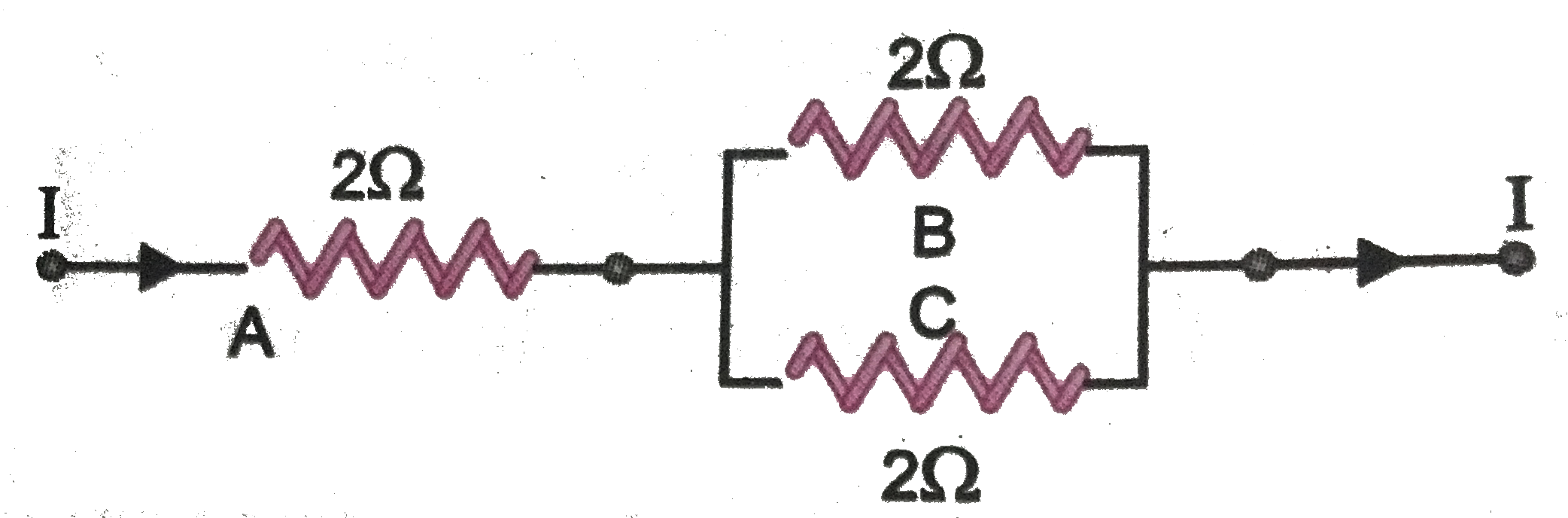# Three 2 Omega reistors , A,B and C, are connected as shown in (Fig. 3.44). Each of them dissipates energy and can withstand a maximum current that

53 views
in Physics
closed
Three 2 Omega reistors , A,B and C, are connected as shown in (Fig. 3.44). Each of them dissipates energy and can withstand a maximum current that can flow through the three resistors ?.

by (71.9k points)
selected by

As P = I^2 R, I = sqrt((P)/(R ))
Thus, maximum current that can flow through 2 Omega resistor (rating 18 W), I = sqrt((18 W)/(2 Omega)) = 3 A.
Since resistor B (= 2 Omega) and resistor C(= 2 Omega) are in parallel, and the current through their combination, which is in series with resistor A(= 2 Omega) is also 3 A, current through B and C (having equal resistance and in parallel) = (3 A)/(2) = 1.5 A.